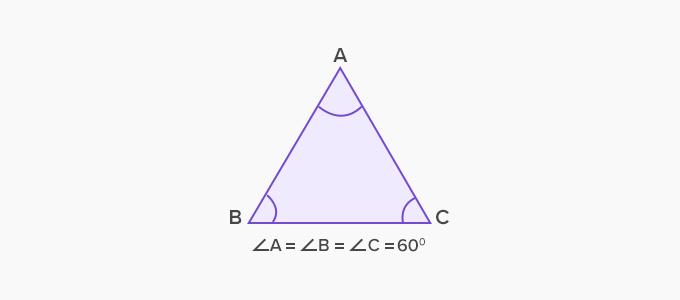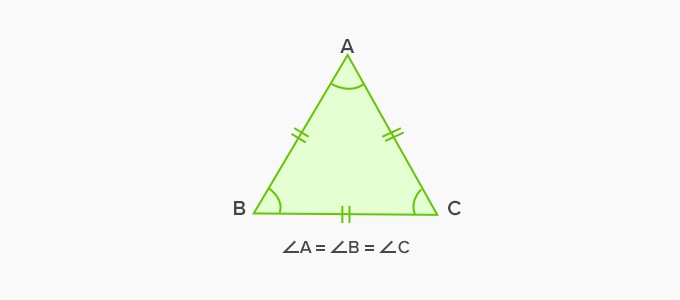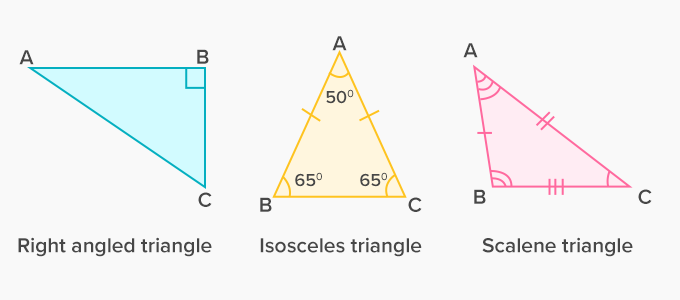# Equiangular Triangle - Definition with Examples

The Complete K-5 Math Learning Program Built for Your Child

• 30 Million Kids

Loved by kids and parent worldwide

• 50,000 Schools

Trusted by teachers across schools

• Comprehensive Curriculum

Aligned to Common Core

What is Equiangular Triangle?

A triangle with three equal interior angles is called an equiangular triangle. In an equiangular triangle, the measure of each of its interior angles is 60 ̊.An equiangular triangle has three equal sides, and it is the same as an equilateral triangle.

Example of equiangular triangle:Non-examples of equiangular triangle:In the given non-examples, all the figures are of triangles, but none of them is an equiangular triangle because:

• In a right-angled triangle, one of the interior angles is of 90˚.

• In an isosceles triangle, only two interior angles are equal.

• In a scalene triangle, none of the interior angles is equal.

For a triangle to be equiangular all its three interior angles must be equal, that is, each angle should measure 60˚.

 Fun Facts The word “equiangular” means “equal angles”. An acute angle triangle is a triangle in which all the three interior angles are less than 90˚. Since, the measure of each of the interior angles of an equiangular triangle is 60˚, an equiangular triangle is always an acute-angled triangle.

Won Numerous Awards & Honors